Math vocabulary. Lessons 6-10Page 1

WATCH ALL SLIDES

Slide 1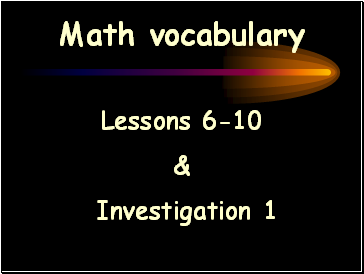Math vocabulary

Lessons 6-10

&

Investigation 1

Slide 2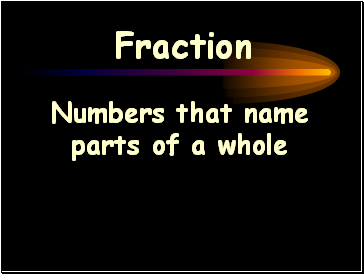Fraction

Numbers that name parts of a whole

Slide 3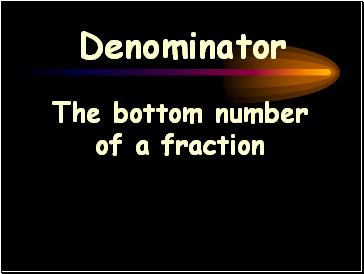Denominator

The bottom number of a fraction

Slide 4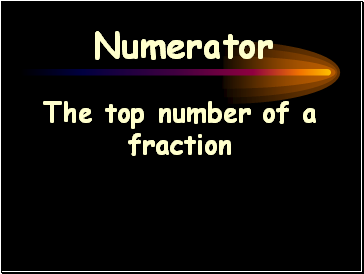Numerator

The top number of a fraction

Slide 5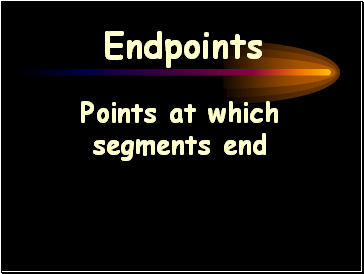Endpoints

Points at which segments end

Slide 6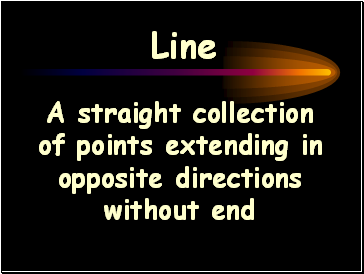Line

A straight collection of points extending in opposite directions without end

Slide 7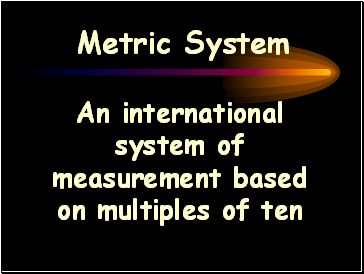Metric System

An international system of measurement based on multiples of ten

Slide 8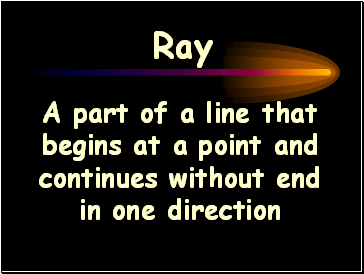Ray

A part of a line that begins at a point and continues without end in one direction

Slide 9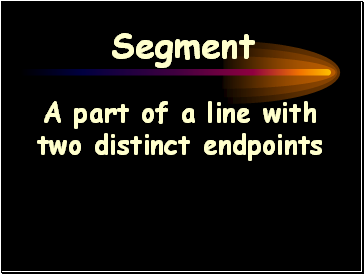Segment

A part of a line with two distinct endpoints

Slide 10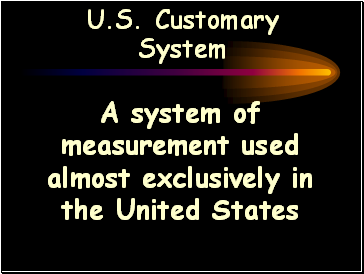U.S. Customary System

A system of measurement used almost exclusively in the United States

Slide 11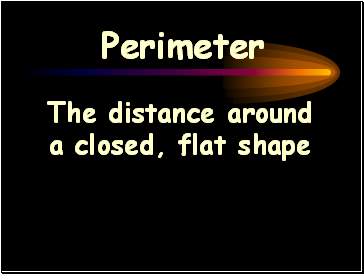Perimeter

The distance around a closed, flat shape

Slide 12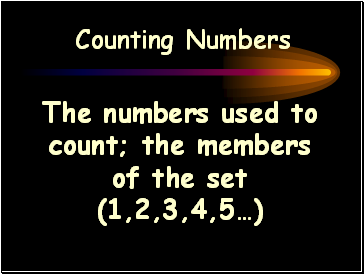Counting Numbers

The numbers used to count; the members of the set (1,2,3,4,5…)

Slide 13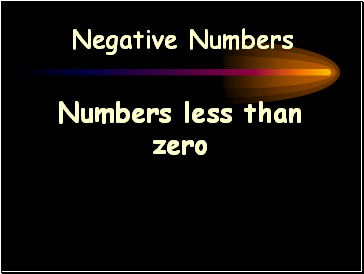Negative Numbers

Numbers less than zero

Slide 14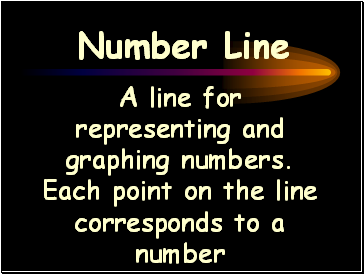Number Line

A line for representing and graphing numbers. Each point on the line corresponds to a number

Slide 15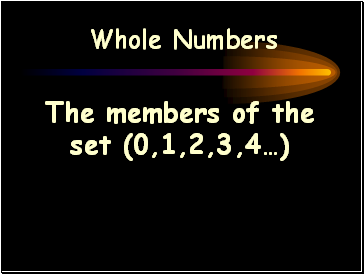Whole Numbers

The members of the set (0,1,2,3,4…)

Slide 16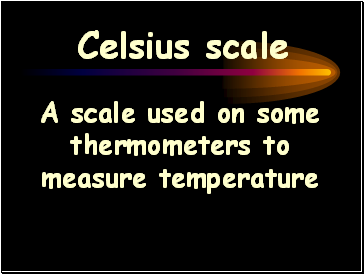Celsius scale

A scale used on some thermometers to measure temperature

Slide 17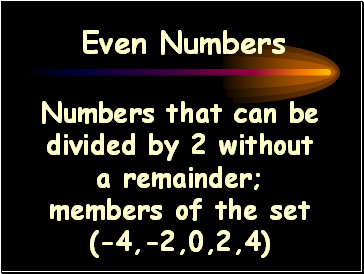Even Numbers

Numbers that can be divided by 2 without a remainder; members of the set (-4,-2,0,2,4)

Go to page:
1  2